The Euclid's algorithmto calculate the Greatest Common Divisor (GCD) between two natural numbers

(R. Bigoni)

The Euclid's algorithm relies on the following theorem:

Given two natural numbers a e b, both greater than 1 and such that a ≥ b:

• if b exactly divides a, b is obviously the GCD of the two numbers;
• else, said r the remainder of the division of a by b, the GCD between a e b is equal to the CGD between b and r.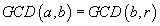Proof

If a is greater than b and isn't multiple of b, said d the GCD(a,b), we have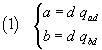where qad and qbd denote respectively the quotients of the divisions of a by d and of b by d.

If we call qab the quotient of the division of a by b and r the remainder of the same division (r < b) we have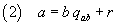If we now substitute in (2) the second sides of (1) we have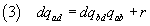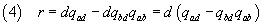therefore d divides also r.

So

• while r > 0 we can replace the calculation of GCD(a,b) with the calculation of GCD(b,r);
• when r becomes 0, then GCD(a,b) coincides with the previous remainder.

The Least Common Multiple (LCM)

Given two natural numbers a e b, both greater than 1,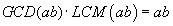Proof

Let d = GCD(a,b) and m = LCM(a,b).

We have

•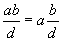is multiple of a
•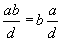is multiple of b
•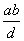is a common multiple of a e b and, obviously, it is or greater than or equal to m: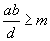;

Therefore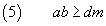We have also

•is a divisor of a. Indeed•is a divisor of b. Indeed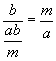•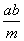is a common divisor of a and b and, obviously, it is less than or equal to d:Therefore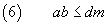(5) and (6) must hold together, so we have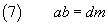Finally, from (7) we havethus

the LCM of two numbers is given by the division of their product by their GCD.

Javascript implementation